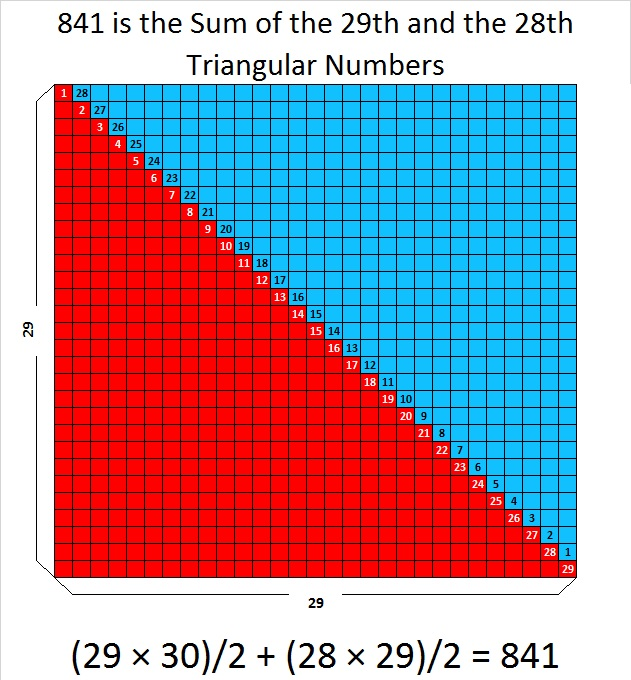# You’ll Be Impressed By 841’s Number Facts

841 is a very cool square number. 29 × 29 = 841. You can write it more compactly: 29² = 841. Here are some facts about this square number:

841 is the sum of the 29th and the 28th triangular numbers because 841 = 435 + 406.What’s that you say? Every other square number can make a similar claim? Oh. … Wait a minute…Not EVERY square number can do that. One is a square number, and it’s NOT the sum of two triangular numbers. So there…

How about this… 841 is the sum of the first 29 odd numbers. That makes it the sum of all the odd numbers from 1 to 57. That’s impressive!What? All square numbers do that? Huh??? n² is always the sum of the first n odd numbers? Why’d you have to tell me that fact isn’t particularly unique either!

Okay…841 is an interesting number in a few other bases. Pay attention….  841 is

• 100 in BASE 29
• 121 in BASE 28
• 144 in BASE 27
• 169 in BASE 26
• 441 in BASE 14

Ha! You didn’t know that one! … Now don’t go spoiling my glee by telling me that those same square numbers show up for a few other square numbers, too. Don’t tell me that!

Give me one more chance to impress you….This square number, 841, is the sum of two consecutive square numbers, so 20² + 21² = 841 = 29². That hasn’t happened to a square number since 3² + 4² = 25 = 5². That makes 841 the 21st Centered Square Number but only the SECOND square number that is both kinds of squares! There may be an infinite number of squares that do the same thing, but it is still a fairly unique characteristic.There are 441 blue squares in that graphic. There are 400 squares that are orange, red, green, purple, or black. 441 + 400 = 841.

Here are a few other tidbits about 841:

841 is the sum of the nine prime numbers from 73 to 109. It is also the sum of three consecutive primes: 277 +  281 + 283 = 841

841 is the hypotenuse of TWO Pythagorean triples:

• 580-609-841 which is 29 times (20-21-29)
• 41-840-841 calculated from 21² – 20², 2(21)(20), 21² + 20²

841 Pythagorean triple Recursion: Hmm…How Quickly Can I Give You a Headache? Warning! Reading this set of bullet points might overload your brain:

• 5² + 2² = 29, so (2∙5∙2)² + (5² – 2²)² = (5² + 2²)² which means 20² + 21² = 29² = 841.
• 21² + 20² = 841, so (2∙21∙20)² + (21² – 20²)² = (21² + 20²)² which means 840² + 41² = 841² = (29²)² = 707281.
• 840² + 41² = 707281, so (2∙840∙41)² + (840² – 41²)² = (840² + 41²)² which means 68880² + 703919² = 707281² = ((29²)²)² = 500,246,412,961
• We could go on forever with even bigger powers of 29 …

We’ll finish with just some simple, easy-on-the-brain facts about 841:

29 is the tenth prime number. Its square, 841, is only the tenth number to have exactly three factors.

• 841 is a composite number.
• Prime factorization: 841 = 29²
• The exponent in the prime factorization is 2. Adding one we get (2 + 1) = 3. Therefore 841 has exactly 3 factors.
• Factors of 841: 1, 29, 841
• Factor pairs: 841 = 1 × 841 or 29 × 29
• 841 is a perfect square. √841 = 29I’m impressed by all this, even if you aren’t.

## 3 thoughts on “You’ll Be Impressed By 841’s Number Facts”

1.Joseph Nebus

You know, the different base representations are really intriguing me.

•ivasallay

That’s what surprised me the most, too.

2.Barbara

Thanks so much

This site uses Akismet to reduce spam. Learn how your comment data is processed.